×#### Thank you for registering.

One of our academic counsellors will contact you within 1 working day.

Click to Chat

1800-1023-196

+91-120-4616500

CART 0

• 0

MY CART (5)

Use Coupon: CART20 and get 20% off on all online Study Material

ITEM
DETAILS
MRP
DISCOUNT
FINAL PRICE
Total Price: Rs.

There are no items in this cart.
Continue Shopping• Complete JEE Main/Advanced Course and Test Series
• OFFERED PRICE: Rs. 15,900
• View Details

Chapter 8: Linear Equations in One Variable Exercise – 8.3

Question: 1

6x + 5 = 2x + 17

Solution:

We have

6x + 5 = 2x+ 17

Transposing 2x to LHS and 5 to RHS, we get

6x – 2x = 17 – 5

4x = 12

Dividing both sides by 4, we get

4x/4 = 12/4

x = 3

Verification:

Substituting x = 3 in the given equation, we get

6 × 3 + 5 = 2 × 3 + 17

18 + 5 = 6 + 17

23 = 23

LHS = RHS

Hence, verified.

Question: 2

2(5x – 3) -3(2x – 1) = 9

Solution:

We have

2(5x – 3) – 3(2x – 1) = 9

Expanding the brackets, we get

2 × 5x – 2 × 3 – 3 × 2x + 3 × 1 = 9

10x – 6 - 6x + 3 = 9

10x – 6x – 6 + 3 = 9

4x – 3 = 9

Adding 3 to both sides, we get

4x – 3 + 3 = 9 + 3

4x = 12

Dividing both sides by 4, we get

4x/4 = 12/4

Thus, x = 3.

Verification:

Substituting x = 3 in LHS, we get

= 2(5 × 3 – 3) – 3(2 × 3 – 1)

= 2 × 12 – 3 × 5

= 24 – 15

= 9

LHS = RHS

Hence, verified.

Question: 3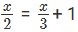Solution: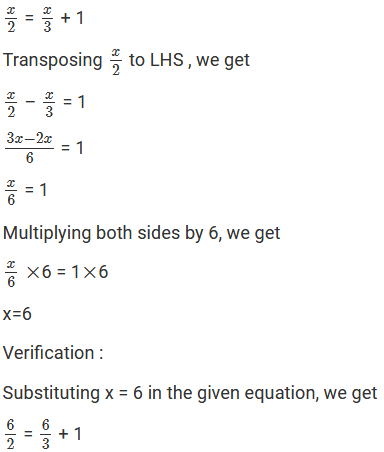3 = 2 + 1

3 = 3

LHS = RHS

Hence, verified.

Question: 4Solution: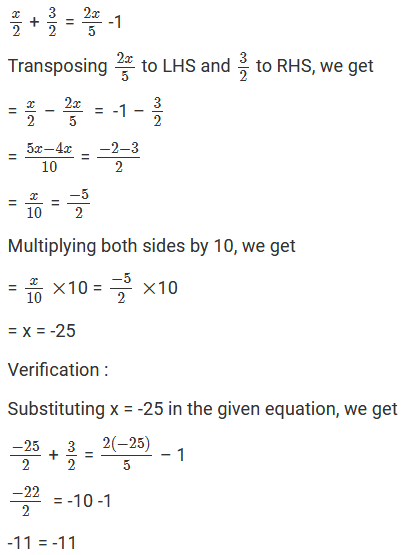LHS = RHS

Hence, verified.

Question: 5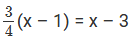Solution:On expanding the brackets on both sides, we get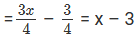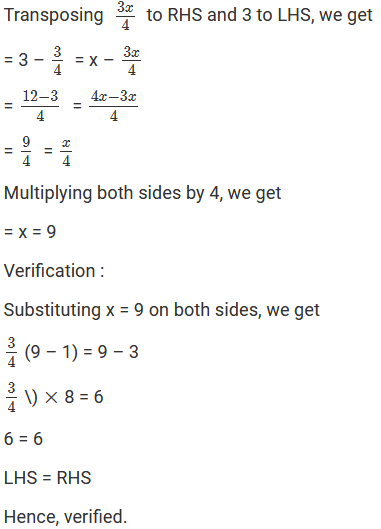Question: 6

3(x – 3) = 5(2x + 1)

Solution:

3(x – 3) = 5(2x + 1)

On expanding the brackets on both sides, we get

= 3 × x – 3 × 3 = 5 × 2x + 5 × 1

= 3x – 9 = 10x + 5

Transposing 10x to LHS and 9 to RHS, we get

= 3x – 10x = 9 + 5

= -7x = 14

Dividing both sides by 7, we get

= –7x/7 = 14/7

=x = -2

Verification:

Substituting x = -2 on both sides, we get

3(-2 – 3) = 5{2(-2) +1}

3(-5) = 5(-3)

-15 = -15

LHS = RHS

Hence, verified.

Question: 7

3x – 2(2x – 5) = 2(x + 3) – 8

Solution:

3x – 2(2x – 5) = 2(x + 3) – 8

On expanding the brackets on both sides, we get

= 3x – 2 × 2x + 2 × 5 = 2 × x + 2 × 3 – 8

= 3x – 4x + 10 = 2x + 6 – 8

= -x + 10 = 2x – 2

Transposing x to RHS and 2 to LHS, we get

= 10 + 2 = 2x + x

= 3x = 12

Dividing both sides by 3, we get

= 3x/3 = 12/3

= x = 4

Verification:

Substituting x = 4 on both sides, we get

3(4) – 2{2(4) – 5} = 2(4 + 3) – 8

12 – 2(8 – 5) = 14 – 8

12 – 6 = 6

6 = 6

LHS = RHS

Hence, verified.

Question: 8Solution:Transposing x/4 to LHS and – (1/2) to RHS, we getMultiplying both sides by 4, we get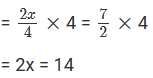Dividing both sides by 2, we get

= 2x/2 = 14/2

= x = 7

Verification:

Substituting x = 7 on both sides, we getQuestion: 9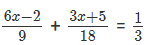Solution: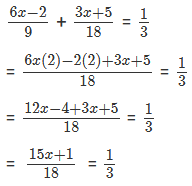Multiplying both sides by 18, we getTransposing 1 to RHS, we get

= 15x = 6 – 1

= 15x = 5

Dividing both sides by 15, we get

= 15x/15 = 5/15

=x = 1/3

Verification :

Substituting x = 1/3 both sides, we get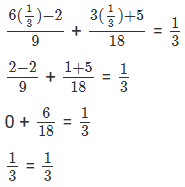LHS = RHS

Hence, verified.

Question: 10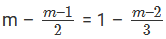Solution: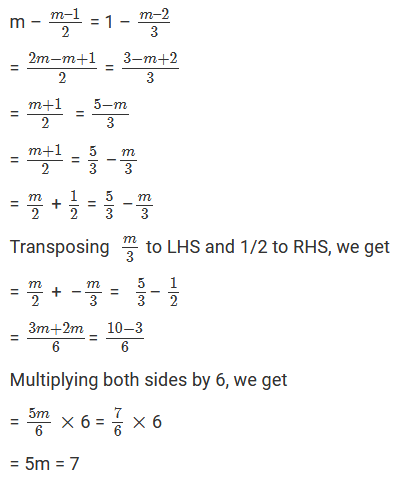Dividing both sides by 5, we get

= 5m/5 = 7/5

= m = 7/5

Verification:

Substituting m = 75 on both sides, we get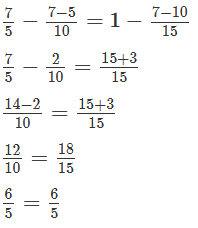LHS = RHS

Hence, verified.

Question: 11Solution: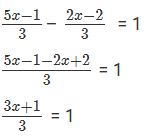Multiplying both sides by 3, we get 3Subtracting 1 from both sides, we get

= 3x + 1 – 1 = 3 – 1

= 3x = 2

Dividing both sides by 3, we get

= 3x + 1 - 1 = 3 -1

= 3x = 2

Dividing both sides by 3, we get

= 3x/3 = 2/3

= x = 2/3

Verification:

Substituting x = 2/3 in LHS, we get

= RHS

LHS = RHS Hence, verified.

Question: 12

0.6x + 4/5 = 0.28x + 1.16

Solution:

0.6x + 4/5 = 0.28x + 1.16

Transposing 0.28x to LHS and 45 to RHS, we get

= 0.6x – 0.28x = 1.16 – 45

= 0.32x = 1.16 – 0.8

= 0.32x = 0.36

Dividing both sides by 0.32, we get

= 0.32 x 0.32 = 0.360.32

= x = 98

Verification:

Substituting x = 98 on both sides, we get

0.6(9/8) + 45 = 0.28(9/8) + 1.16

5.4/8 + 4/5 = 2.52/8 + 1.16

0.675 + 0.8 = 0.315 + 1.16

1.475 = 1.475

LHS = RHS

Hence, verified.

Question: 13

0.5x + x/3 = 0.25x + 7

Solution:

0.5x + x/3 = 0.25x + 7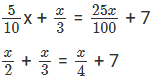Transposing x/4 to LHS, we get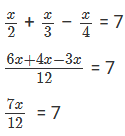Multiplying both sides by 12, we get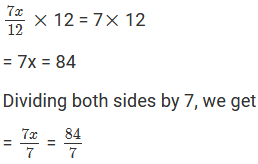= x = 12

Verification:

Substituting x = 12 on both sides, we get

0.5(12) + (12)3 = 0.25(12) + 7

6 + 4 = 3 + 7

10 = 10

LHS = RHS

Hence, verified.### Course Features

• 728 Video Lectures
• Revision Notes
• Previous Year Papers
• Mind Map
• Study Planner
• NCERT Solutions
• Discussion Forum
• Test paper with Video Solution